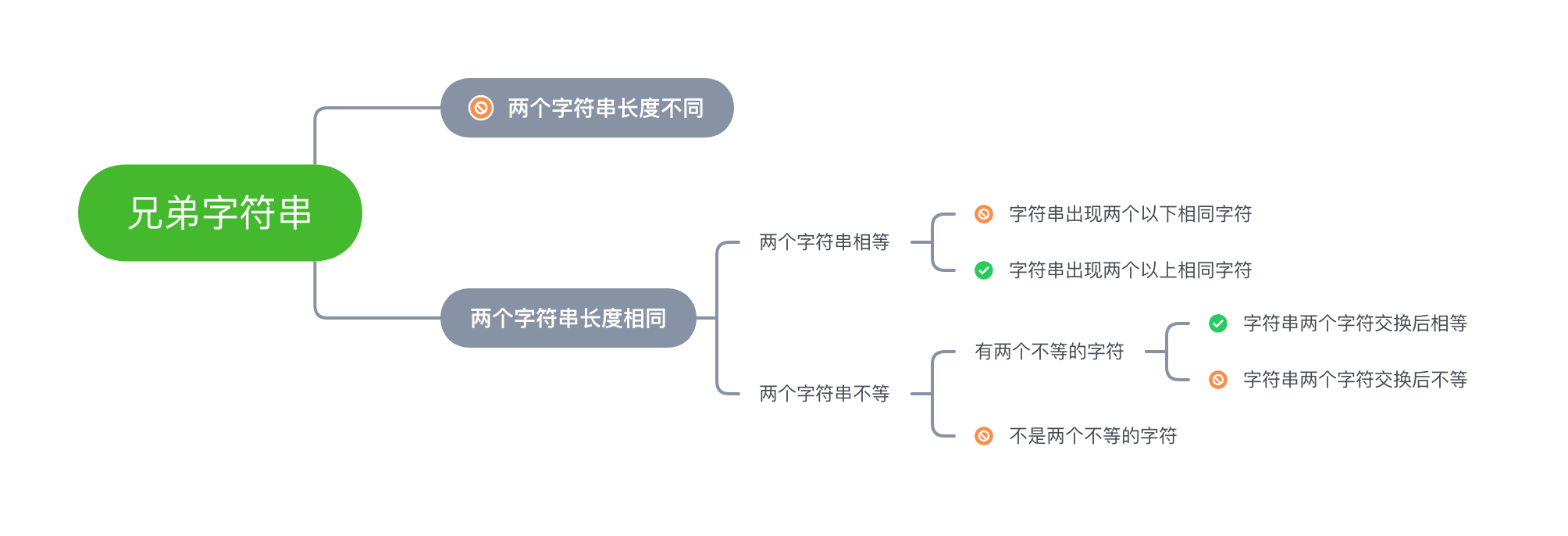[leetcode 859] 亲密字符串

### Problem:

859. 亲密字符串

``````输入： A = "ab", B = "ba"

``````

``````输入： A = "ab", B = "ab"

``````

``````输入： A = "aa", B = "aa"

``````

``````输入： A = "aaaaaaabc", B = "aaaaaaacb"

``````

``````输入： A = "", B = "aa"

``````

1. `0 <= A.length <= 20000`
2. `0 <= B.length <= 20000`
3. `A``B` 仅由小写字母构成。

### Solution:``````func buddyStrings(A string, B string) bool {
isBuddyStrings := true
var diffA []string
var diffB []string
var isSameWordExistedString bool

// 如果两个字符串长度不同，不是兄弟字符串
if len(diffA) != len(diffB) {
return false
}

tempStrMap := make(map[string]bool)
for i := 0; i < len(A); i++ {
// 每个位置不相同的字符
if A[i] != B[i] {
diffA = append(diffA, string(A[i]))
diffB = append(diffB, string(B[i]))
}

// 判断有没有出现两个以上相同字符
_, existed := tempStrMap[string(A[i])]
if existed {
isSameWordExistedString = true
}
tempStrMap[string(A[i])] = true
}

// 如果两个字符串相同，判断是否包含相同字母
if len(diffA) == 0 && len(diffB) == 0 {
if isSameWordExistedString {
return true
}

return false
}

// 如果两个字符串不相同，判断是不是有且只有两个不同字符
if len(diffA) != 2 && len(diffB) != 2 {
return false
}

// 如果两个字符串不相同，判断交换后的字符是不是相同
if diffA != diffB || diffA != diffB {
return false
}

return isBuddyStrings
}

``````

If You Have Any Question, You Can Contact Me Through liam@blue7wings.com, @Blue7Wings, #Liam_Hsia